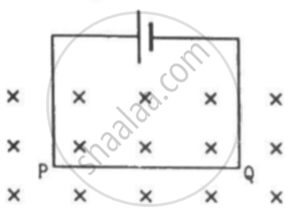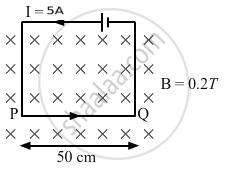Department of Pre-University Education, KarnatakaPUC Karnataka Science Class 12
Advertisement Remove all ads

# A Current of 5.0 a Exists in the Circuit Shown in the Figure. the Wire Pq Has a Length of 50 Cm and the Magnetic Field in Which It is Immersed Has a Magnitude - Physics

Sum

A current of 5.0 A exists in the circuit shown in the figure. The wire PQ has a length of 50 cm and the magnetic field in which it is immersed has a magnitude of 0.20 T. Find the magnetic force acting on the wire PQ.Advertisement Remove all ads

#### Solution

Given:
Length of the wire PQ inside the magnetic field, = 50 cm
Electric current through the wire, I = 5 A
Magnetic field, B = 0.2 T
The direction of magnetic field is perpendicular to the plane of the frame and it is going into the plane of the circuit.
As per the question,Angle between the plane of the wire and the magnetic field, θ = 90˚
Magnetic force,
vecF = vecil xx vecB
therefore |vecF| = ilBsintheta
therefore |vecF| = ilBsin906circ
= 5 × 50 × 10−2 × 0.2 × 1
= 0.50 N
The direction of force can be found using Fleming's left-hand rule.
Thus, the direction of magnetic force is upwards in the plane of the paper.

Concept: Force on a Current - Carrying Conductor in a Uniform Magnetic Field
Is there an error in this question or solution?
Advertisement Remove all ads

#### APPEARS IN

HC Verma Class 11, Class 12 Concepts of Physics Vol. 2
Chapter 12 Magnetic Field
Q 11 | Page 231
Advertisement Remove all ads

#### Video TutorialsVIEW ALL 

Advertisement Remove all ads
Share
Notifications

View all notifications

Forgot password?